Polynomial fit passing through specified points

Jiro's pick this week is polyfix by Are Mjaavatten.

Have you ever wanted to fit a polynomial to your data and have the line go through some specified points? What about specifying the slope at a certain point? Let's take a look at some options, including Are's entry.

Contents

Polynomial fitting

The function polyfit lets you fit a polynomial to your data. We'll first create some data.

t = linspace(0,2,31)';
y = sin(pi*t)+ 0.1*randn(size(t));
plot(t,y,'o')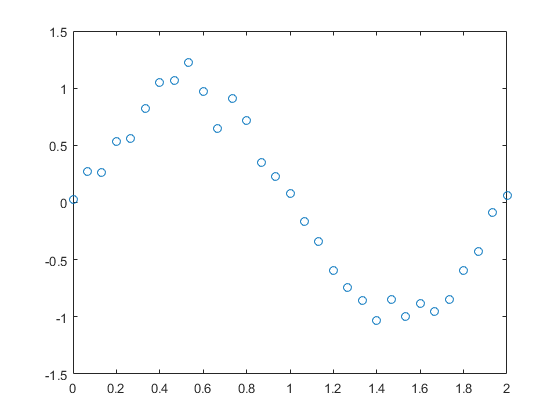We'll fit a 3rd order polynomial to the data.

$$y \approx x_1t^3 + x_2t^2 + x_3t + x_4$$

x_polyfit = polyfit(t,y,3)
y1 = polyval(x_polyfit,t);
plot(t,y,'o',t,y1)
legend('data','polyfit')
x_polyfit =
2.8843   -8.6941    6.0419   -0.2050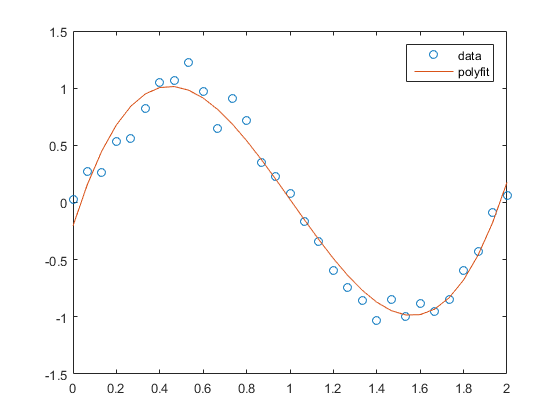Constrain to go through certain points

What if you want this polynomial to go through certain points. Perhaps, you want the curve to cross (0, 0) and (2, 0). This is where Are's entry comes into play. But first, let me talk about a different method. I found this question on MATLAB Answers. There are several ways to deal with this, and one of them is to use a function like lsqlin from Optimization Toolbox. lsqlin solves the following least-squares curve fitting problem.

$$\min_{x}\frac{1}{2}||C\cdot x - d||_2^2 \quad \rm {such \; that} \quad \Bigg \{ \begin{array}{l} A \cdot x \leq b, \\ Aeq \cdot x = beq, \\ lb \leq x \leq ub \end{array}$$

For a 3rd order polynomial, $C$ and $d$ are defined as the following.

$$C = \left[ t^3 \quad t^2 \quad t \quad 1 \right],\quad d = y$$

$x$ represents the coefficients for the polynomial terms.

$$x : \left[ \begin{array}{c} x_1 \\ x_2 \\ x_3 \\ x_4 \end{array} \right]$$

C = [t.^3 t.^2 t ones(size(t))];
d = y;

There are no inequality constraints, so we set $A$ and $b$ to empty.

A = [];     % No inequality constraint
b = [];     % No inequality constraint

For the equality constraints, we need to set the $y$ values at $t$ = 0 and $t$ = 2 to zero. This means

$$\begin{array}{rccccc} x_1(0)^3 + x_2(0)^2 + x_3(0) + x_4 = 0 & \Rightarrow & [0\quad0\quad0\quad1] & \cdot & x = & 0 \\ x_1(2)^3 + x_2(2)^2 + x_3(2) + x_4 = 0 & \Rightarrow & \underbrace{[2^3\quad2^2\quad2\quad1]}_{Aeq} & \cdot & x = & \underbrace{0}_{beq} \end{array}$$

Putting these into $Aeq$ and $beq$, we get the following.

Aeq = [0    0    0    1 ;   % t = 0
2^3  2^2  2    1];   % t = 2
beq = [0 ;                  % y = 0 (when t = 0)
0];                  % y = 0 (when t = 2)

We then call lsqlin to solve for the polynomial coefficients.

x_lsqlin1 = lsqlin(C,d,A,b,Aeq,beq)
Warning: The trust-region-reflective algorithm can handle bound constraints
only;
Optimization terminated.
x_lsqlin1 =
2.5524
-7.6807
5.1518
0
plot(t,y,'o',t,C*x_lsqlin1)
legend('data','lsqlin')
grid on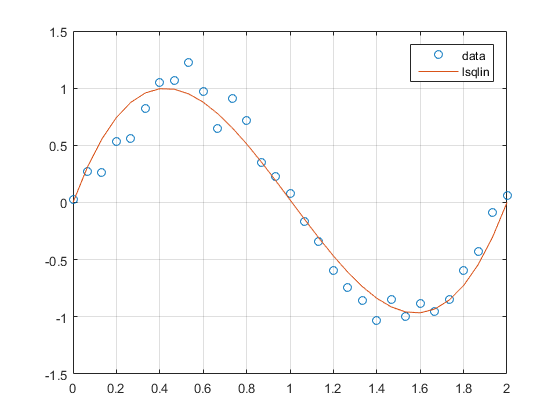Constrain to have a certain derivative

Let's add more constraint for the curve to go through (0.5,1) and (1.5,-1). Also, we want the derivatives at those points to be zero. That's additional 4 constraints. With so many constraints, we won't be able to satisfy them with a 3rd order polynomial. Let's try a 7th order.

$$y \approx x_1t^7 + x_2t^6 + x_3t^5 + x_4t^4 + x_5t^3 + x_6t^2 + x_7t + x_8$$

C = [t.^7 t.^6 t.^5 t.^4 t.^3 t.^2 t ones(size(t))];

The derivative for the 7th order polynomial is defined as

$$\frac{dy}{dt} \approx 7x_1t^6 + 6x_2t^5 + 5x_3t^4 + 4x_4t^3 + 3x_5t^2 + 2x_6t + x_7$$

For example, the constraint "the derivative at $t$ = 0.5 is zero" can be represented as

$$\begin{array}{c} { 7x_1(0.5)^6 + 6x_2(0.5)^5 + 5x_3(0.5)^4 + 4x_4(0.5)^3 + 3x_5(0.5)^2 + 2x_6(0.5) + x_7 = 0 } \\ \\ { \Downarrow } \\ \\ { \underbrace{\left[ 7(0.5)^6\quad6(0.5)^5\quad5(0.5)^4\quad4(0.5)^3\quad3(0.5)^2\quad2(0.5)\quad1\quad0\right]}_{Aeq}\cdot x = \underbrace{0}_{beq} } \end{array}$$

Aeq = [0       0       0       0       0       0     0     1 ;   % t = 0
2^7     2^6     2^5     2^4     2^3     2^2   2     1 ;   % t = 2
0.5^7   0.5^6   0.5^5   0.5^4   0.5^3   0.5^2 0.5   1 ;   % t = 0.5
1.5^7   1.5^6   1.5^5   1.5^4   1.5^3   1.5^2 1.5   1 ;   % t = 1.5
7*0.5^6 6*0.5^5 5*0.5^4 4*0.5^3 3*0.5^2 2*0.5 1     0 ;   % t = 0.5 (for dy/dt)
7*1.5^6 6*1.5^5 5*1.5^4 4*1.5^3 3*1.5^2 2*1.5 1     0];   % t = 1.5 (for dy/dt)
beq = [ 0 ;     % y = 0 (when t = 0)
0 ;     % y = 0 (when t = 2)
1 ;     % y = 1 (when t = 0.5)
-1 ;     % y = -1 (when t = 1.5)
0 ;     % dy/dt = 0 (when t = 0.5)
0];     % dy/dt = 0 (when t = 1.5)

Let's fit and see if we've accomplished our goal.

x_lsqlin2 = lsqlin(C,d,A,b,Aeq,beq)
plot(t,y,'o',t,C*x_lsqlin2)
legend('data','lsqlin (with derivative constraint)')
grid on
set(gca,'XTick',0:0.5:2)   % Adjust tick marks to show points of interest
Warning: The trust-region-reflective algorithm can handle bound constraints
only;
Optimization terminated.
x_lsqlin2 =
-0.2925
2.1030
-7.8142
17.6810
-19.6794
5.7234
2.2753
0.0000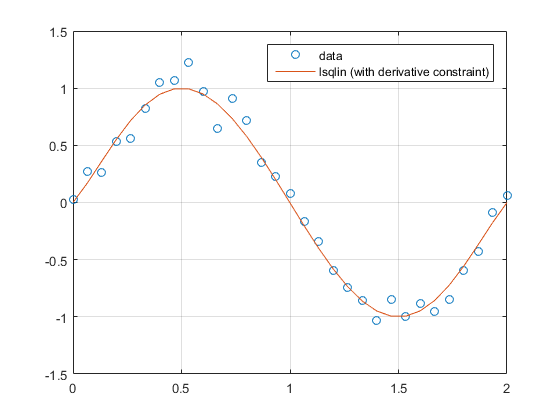polyfix

In the MATLAB Answers post I mentioned above, Are actually posted a response mentioning polyfix. This entry achieves the goal of performing a polynomial fit with constraints to pass through specific points with specific derivatives. Let's solve the same problem using polyfix, 7th order polynomial to fit through (0,0), (2,0), (0.5,1), (1.5,-1) and derivative of zero at $t$ = 0.5 and $t$ = 1.5.

x_polyfix = polyfix(t,y,7,[0 2 0.5 1.5],[0 0 1 -1],[0.5 1.5],[0 0])
plot(t,y,'o',t,polyval(x_polyfix,t))
legend('data','polyfix')
grid on
set(gca,'XTick',0:0.5:2)   % Adjust tick marks to show points of interest
x_polyfix =
Columns 1 through 7
-0.2925    2.1030   -7.8142   17.6810  -19.6794    5.7234    2.2753
Column 8
0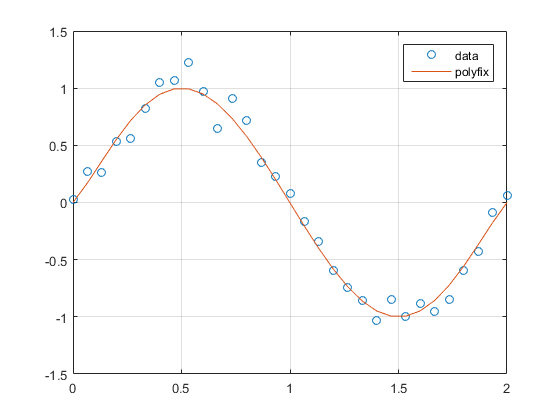If we compare this result with that from lsqlin, we see that it's essentially identical.

norm(x_lsqlin2-x_polyfix')
ans =
2.1194e-10

Optimization Toolbox

Are's polyfit is great for performing polynomial fits with constraints around passing points. If you need other sophisticated constraints, you would want to check out Optimization Toolbox.